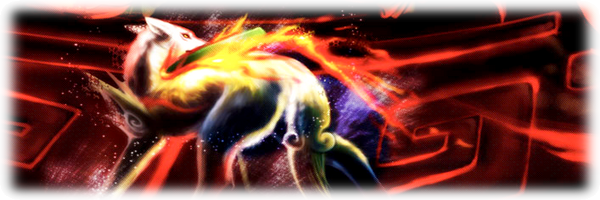Help for Ghost Trick NDS

Une question en rapport avec le ROMHacking ?
Dans cette section nous tenterons de vous conseiller ou de partager nos connaissances, bien entendu dans la limite de nos compétences et de notre disponibilité.

Help for Ghost Trick NDS

Lyan53 a écrit:
cierpa a écrit:Ummm... I have a question. Does anyone here trying to hack .xml files from Ghost Trick?

This game is release in french language, I suppose nobody's try to hack it in FranceBut I see inside .XML files and that is what I found :

.xml.lz files are compressed in Lzss type 11 (use batch lz77 or lzss codec for extract it)
.xml.bin files are not compressed

Inside, I found game script with relative search:And the character table (not complete):
Code: Tout sélectionner
`0A00=A0B00=B0C00=C0D00=D0E00=E0F00=F1000=G1100=H1200=I1300=J1400=K1500=L1600=M1700=N1800=O1900=P1A00=Q1B00=R1C00=S1D00=T1E00=U1F00=V2000=W2100=X2200=Y2300=Z2400=a2500=b2600=c2700=d2800=e2900=f2A00=g2B00=h2C00=i2D00=j2E00=k2F00=l3000=m3100=n3200=o3300=p3400=q3500=r3600=s3700=t3800=u3900=v3A00=w3B00=x3C00=y3D00=z4500=Ç5900=à5B00=â5E00=ç5F00=è6000=é6100=ê6500=î7000=ûE100=.EA00="EF00=,F300='FF00= 01FF=<line??>03FF=<begin??>`

It seems .xml files contains several sub-files (the name of sub-file are stocked in the end of .xml)

My search are make on the "st01_game000_Expand.fr.xml" file ("data /st01/" folder)

In the header of .xml files :In green >> File ID
In red >> size of game script table >> offset 0x34 to 0x2977
In orange >> ?? (I don't know)
In blue > size of sub-files index + files names >> offset 0x2978 to the end of file ( - 0x4 bytes of padding)

Detail for sub-files index:

In green > I'm not sure, maybe indicate a begin of new data table (0x2A for "index table" and 0x2A too for "names table")
In orange > number of files stocked inside index

In this index, each file is stocked in 0x8 bytes:
In red > offset relative to name table (name of file) >> beginning of this table on offset 0x2978
In blue > offset relative to game script

Good luckLyan53

Messages: 864
Âge: 41
Enregistré le: Lundi 22 Novembre 2010 à 20:48:11
Genre:Retourner vers Sur le ROMhacking

Qui est en ligne

Utilisateurs parcourant ce forum : Aucun utilisateur enregistré et 1 invité

x

#{text}